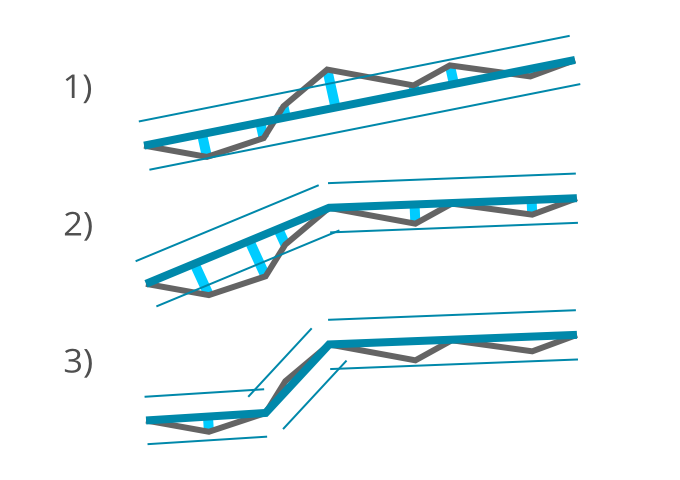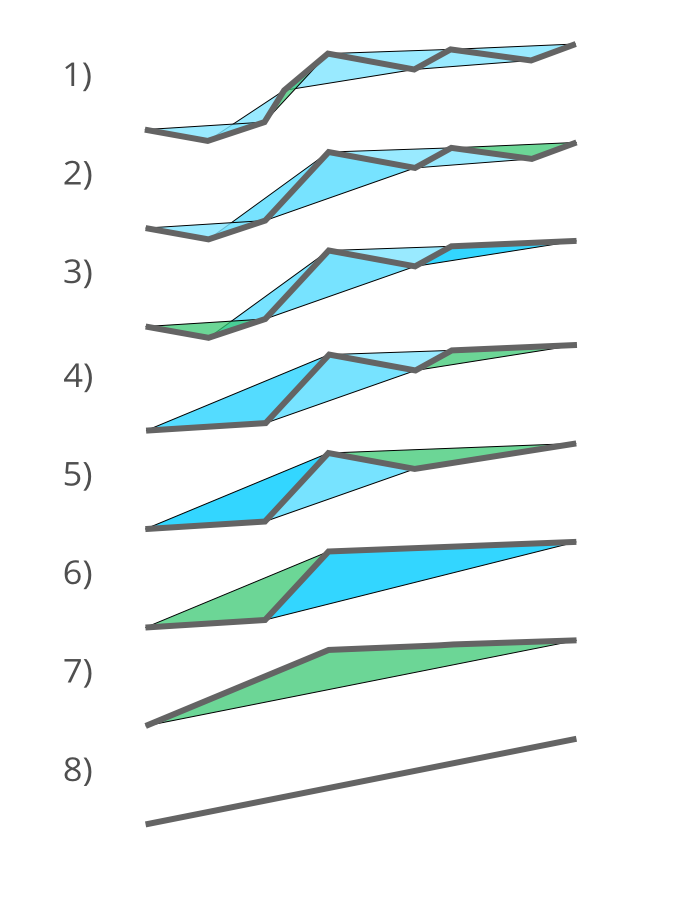# Polyline Simplification

Polylines (and polygons) often have too many points in them for a particular application. For example, a coastline would not need to have meter accuracy if it was being printed at a world map scale. Topography contours taken from a DEM could have so many points that they cause downstream processes to be sluggish and unresponsive. There are many other reasons that we would like to reduce the number of points in a polyline. Several algorithms exist for this purpose.

One of the first, and most well known, algorithms for polyline simplificiation is the Ramer Douglas Peucker (RDP) algorithm. This algorithm was independently developed by both Urs Ramer, 1972, and David Douglas and Thomas Peucker, 1973, in the early 1970s. This algorithm is recursive, it operates by re-introducing points which are greater than some user defined distance away from the current segment. This is what was originally in place, but there are actually a lot of times that I don't like this one.

An alternative algorithm is the Visvalingam Whyatt algorithm. Published by M. Visvalingam and J.D. Whyatt in 2013. This algorithm operates in a fundamentally different way than the RDP algorithm. Instead of re-introducing points, it operates by removing points which have the smallest triangular area and the smallest impact on the overall shape of the line.

I implemented both of these algorithms, and a few others, to be included as a part of the CAD tools in Vulcan. Of course Vulcan already *had* some filtering tools, but it didn't have the Visvalingam Whyatt algorithm, and the RDP filter was less efficient than my version.

## Ramer Douglas Peucker Algorithm

The RDP algorithm initially throws everything away except the two end points, then it looks for points that are farther away from the segment than some tolerance. If a point is far enough away, the algorithm introduces that point and recurses on the new segments.

In the figure below we originally approximate the grey polyline with the thick blue polyline consisting of just the endpoints. Then the distances from each point to the simplified polyine are calculated. One of them is father away than the given tolerance, so we reintroduce it. Now we repeat the process for the two new segments. The left segment finds two points which are farther away than the tolerance, so the farthest is reintroduced. At this stage, all points are closer than the tolerance so the algorithm terminates.The Ramer Douglas Peucker algorithm tends to honor deviations. At each iteration it introduces the point which is farthest away from the current segment, even if that point might not be the best choice. This can lead to results which do not seem correct.

Here is an simplistic implementation of the Ramer Douglas Peucker algorithm. This demonstrates the character of the algorithm, it is not the optimized variant that I implemented for work. Some details, and all error handling code are omitted.

```struct Point {
double x, y;
};

// Return the distance between the segment defined by a--b and the point c
double SegmentPointDistance(const Point& a, const Point& b, const Point& c);

// Recursive application of the Ramer Douglas Peucker algorithm
void RDPFilterRec(const std::vector& polyline, double tolerance,
size_t begin, size_t end, std::vector* results) {
// If we have recursed to a segment of two points, then return
if (end - begin <= 2) {
results->push_back(polyline[begin]);
return;
}

// Find the point which is farthest away from the current segment
double max_distance = 0;
size_t split_index = begin;

for (size_t i = begin + 1; i < end - 1; i++) {
double d = SegmentPointDistance(polyline[begin], polyline[end - 1],
polyline[i]);

if (d > max_distance) {
split_index = i;
max_distance = d;
}
}

// Recurse if required
if (max_distance > tolerance) {
RDPFilterRec(polyline, tolerance, begin, split_index + 1, results);
RDPFilterRec(polyline, tolerance, split_index, end, results);
} else {
results->push_back(polyline[begin]);
}
}

// Ramer Douglas Peucker filter on the input polyline with the given tolerance
void RDPFilter(const std::vector& polyline, double tolerance,
std::vector* results) {
RDPFilterRec(polyline, tolerance, 0, polyline.size(), results);
results->push_back(polyline.back());
}
```

One interesting aspect of this implementation is that due to how the recursion proceeds from beginning to end of the result polygon, a simple vector for the result is appropriate. Of course the size of the result polyline is unknown, it depends on the input tolerance and the shape of the input line, but this implementation can still be efficient with respect to memory allocations and copying stuff around.

## Visvalingam Whyatt Algorithm

The Visvalingam Whyatt algorithm is, in my opinion, a real treat. It calculates an effective area for each point which is then used to simplify the polyline. After each point's effective area is calculated all the points below a given area can be efficiently removed in a single pass through the data.

First we consider a triangle for each point, excluding the first and last point, and sort them by area. The triangle with the smallest area is removed, along with its middle point, this sets the effective area for the middle point to the smallest area. The two neighboring triangles are removed, and two new triangles are introduced. We operate in this manner, progressively removing the smallest triangle and assigning the effective area to each point. The effective area never decreases, but does increase as the triangles get bigger.

In the figure below we first calculate the triangle areas for each point. The smallest one is removed, and its effective area is recorded. Once this point is gone it's clear that we need to remove two triangles and introduce two more. We continue to remove the smallest triangle until only the straight line segment between the first and last points is left. Once the effective areas are recorded any level of simplfication can be found in a single pass through the data.The Visvalingam Whyatt algorithm tends to remove deviations from the input line, instead of ensuring that they remain. On most real world datasets it can remove a large proportion of points before it even becomes noticable. Topographies and coastlines are especially amenable to the Visvalingam Whyatt algorithm.

Here is a brief overview of one possible implementation. I first construct a vector of triangle structs which contain their area, and the indices of the points which make them up. Then form a min-heap of these triangle objects by area. The min-heap is an ideal data structure as the smallest triangle can be found very quickly, and as new triangles are added it can be updated efficiently.

Then begin popping off the minimum area triangle, and populate the effective area. Instead of trying to re-prioritise the two triangles which have been invalidated, I took an idea from many implementations of Djikstra's algorithm, which commonly leaves things in the queue, and I only discard the triangles as they become the minimum. As each triangle is removed, two new triangle objects are then added and pushed into the heap.

This implementation can be very quick, and once the effective areas are populated, can simplify large polylines instantly. This makes the algorithm very appropriate for an interactive simplfication which can be user controlled.

## Conclusion

This was a very short, but enjoyable project. I enjoyed manipulating the recursion in the Ramer Douglas Peucker algorithm to effectively stream out the result polyline. Also, the Visvalingam Whyatt algorithm has received a lot of positive feedback, in part due to its speed, but also due to its slightly more applicable results with topography contours.

## References

Douglas, D. H., & Peucker, T. K. (1973). Algorithms for the reduction of the number of points required to represent a digitized line or its caricature. Cartographica: The International Journal for Geographic Information and Geovisualization, 10(2), 112–122.

Ramer, U. (1972). An iterative procedure for the polygonal approximation of plane curves. Computer Graphics and Image Processing, 1(3), 244–256.

Visvalingam, M., & Whyatt, J. (2013). Line generalisation by repeated elimination of points. The Cartographic Journal.